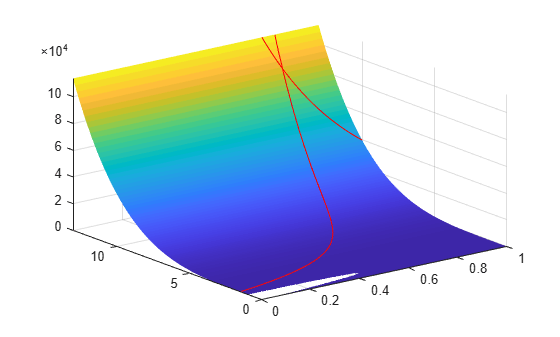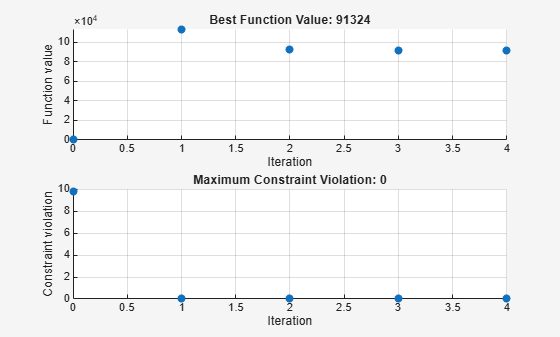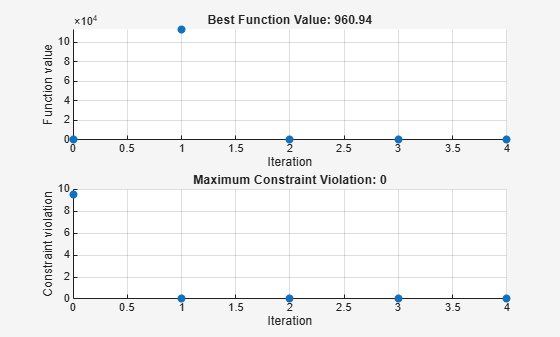# Constrained Minimization Using Pattern Search, Problem-Based

This example shows how to minimize an objective function, subject to nonlinear inequality constraints and bounds, using pattern search in the problem-based approach. For a solver-based version of this problem, see Constrained Minimization Using Pattern Search, Solver-Based.

### Constrained Minimization Problem

For this problem, the objective function to minimize is a simple function of 2-D variables `X` and `Y`:

`camxy = @(X,Y)(4 - 2.1.*X.^2 + X.^4./3).*X.^2 + X.*Y + (-4 + 4.*Y.^2).*Y.^2;`

This function is known as "cam," as described in L.C.W. Dixon and G.P. Szego .

Additionally, the problem has nonlinear constraints and bounds.

``` x(1)*x(2) + x(1) - x(2) + 1.5 <= 0 (nonlinear constraint) 10 - x(1)*x(2) <= 0 (nonlinear constraint) 0 <= x(1) <= 1 (bound) 0 <= x(2) <= 13 (bound) ```

Plot the nonlinear constraint region on a surface plot of the objective function. The constraints limit the solution to the small region above both red curves.

```x1 = linspace(0,1); y1 = (-x1 - 1.5)./(x1 - 1); y2 = 10./x1; [X,Y] = meshgrid(x1,linspace(0,13)); Z = camxy(X,Y); surf(X,Y,Z,"LineStyle","none") hold on z1 = camxy(x1,y1); z2 = camxy(x1,y2); plot3(x1,y1,z1,'r-',x1,y2,z2,'r-') xlim([0 1]) ylim([0 13]) zlim([0,max(Z,[],"all")]) hold off```### Create Optimization Variables, Problem, and Constraints

To set up this problem, create optimization variables `x` and `y`. Set the bounds as you create the variables.

```x = optimvar("x","LowerBound",0,"UpperBound",1); y = optimvar("y","LowerBound",0,"UpperBound",13);```

Create the objective as an optimization expression.

`cam = camxy(x,y);`

Create an optimization problem with this objective function.

`prob = optimproblem("Objective",cam);`

Create the two nonlinear inequality constraints, and include them in the problem.

```prob.Constraints.cons1 = x*y + x - y + 1.5 <= 0; prob.Constraints.cons2 = 10 - x*y <= 0;```

Review the problem.

`show(prob)`
``` OptimizationProblem : Solve for: x, y minimize : (((((4 - (2.1 .* x.^2)) + (x.^4 ./ 3)) .* x.^2) + (x .* y)) + (((-4) + (4 .* y.^2)) .* y.^2)) subject to cons1: ((((x .* y) + x) - y) + 1.5) <= 0 subject to cons2: (10 - (x .* y)) <= 0 variable bounds: 0 <= x <= 1 0 <= y <= 13 ```

### Set Initial Point and Solve

Set the initial point as a structure with field `x` equal to `0.5` and `y` equal to `0.5`.

```x0.x = 0.5; x0.y = 0.5;```

Solve the problem specifying the `patternsearch` solver.

`[sol,fval] = solve(prob,x0,"Solver","patternsearch")`
```Solving problem using patternsearch. Optimization finished: mesh size less than options.MeshTolerance and constraint violation is less than options.ConstraintTolerance. ```
```sol = struct with fields: x: 0.8122 y: 12.3122 ```
```fval = 9.1324e+04 ```

`patternsearch` finds the solution point `x = 0.8122`, `y = 12.3122` with objective function value `9.1324e4`.

To observe the solver's progress, specify options that select two plot functions. The plot function `psplotbestf` plots the best objective function value at every iteration, and the plot function `psplotmaxconstr` plots the maximum constraint violation at every iteration. Set these two plot functions in a cell array. Also, display information about the solver's progress in the Command Window by setting the `Display` option to `'iter'`.

```options = optimoptions(@patternsearch,... "PlotFcn",{@psplotbestf,@psplotmaxconstr},... "Display","iter");```

Run the solver, including the `options` argument.

`[sol,fval] = solve(prob,x0,"Solver","patternsearch","Options",options)`
```Solving problem using patternsearch. Max Iter Func-count f(x) Constraint MeshSize Method 0 1 0.373958 9.75 0.9086 1 18 113581 1.617e-10 0.001 Increase penalty 2 147 92267 0 1e-05 Increase penalty 3 373 91333.2 0 1e-07 Increase penalty 4 638 91324 0 1e-09 Increase penalty Optimization finished: mesh size less than options.MeshTolerance and constraint violation is less than options.ConstraintTolerance. ``````sol = struct with fields: x: 0.8122 y: 12.3122 ```
```fval = 9.1324e+04 ```

Nonlinear constraints cause `patternsearch` to solve many subproblems at each iteration. As shown in both the plots and the iterative display, the solution process has few iterations. However, the `Func-count` column in the iterative display shows many function evaluations per iteration. Both the plots and the iterative display show that the initial point is infeasible, and that the objective function is low at the initial point. During the solution process, the objective function value initially increases, then decreases to its final value.

### Unsupported Functions

If your objective or nonlinear constraint functions are not Supported Operations for Optimization Variables and Expressions, use `fcn2optimexpr` to convert them to a form suitable for the problem-based approach. For example, suppose that instead of the constraint $xy\ge 10$ you have the constraint ${I}_{1}\left(x\right)+{I}_{1}\left(y\right)\ge 10$, where ${I}_{1}\left(x\right)$ is the modified Bessel function `besseli(1,x)`. (The Bessel functions are not supported functions.) Create this constraint using `fcn2optimexpr` as follows. First create an optimization expression for ${I}_{1}\left(x\right)+{I}_{1}\left(y\right)$.

`bfun = fcn2optimexpr(@(t,u)besseli(1,t) + besseli(1,u),x,y);`

Next, replace the constraint `cons2` with the constraint `bfun >= 10`.

`prob.Constraints.cons2 = bfun >= 10;`

Solve the problem. The solution differs because the constraint region is different.

`[sol2,fval2] = solve(prob,x0,"Solver","patternsearch","Options",options)`
```Solving problem using patternsearch. Max Iter Func-count f(x) Constraint MeshSize Method 0 1 0.373958 9.484 0.9307 1 18 113581 1.659e-08 0.001 Increase penalty 2 183 962.841 0 1e-05 Increase penalty 3 499 960.942 0 1e-07 Increase penalty 4 636 960.94 0 8.511e-15 Update multipliers Optimization finished: mesh size less than options.MeshTolerance and constraint violation is less than options.ConstraintTolerance. ``````sol2 = struct with fields: x: 0.4998 y: 3.9981 ```
```fval2 = 960.9401 ```

### References

 Dixon, L. C. W., and G .P. Szego (eds.). Towards Global Optimisation 2. North-Holland: Elsevier Science Ltd., Amsterdam, 1978.